# A 2.8 ?? block attached to a massless spring and is displaced 35 ?? by a...

A 2.8 ?? block attached to a massless spring and is displaced 35 ?? by a force of 927.5 ? across a smooth floor. The box is then let go and undergoes simple harmonic motion. Determine, a. The maximum acceleration of the block. b. The period of oscillation. c. The angular frequency. d. The speed at equilibrium. e. The velocity at ? = 16.2 ?�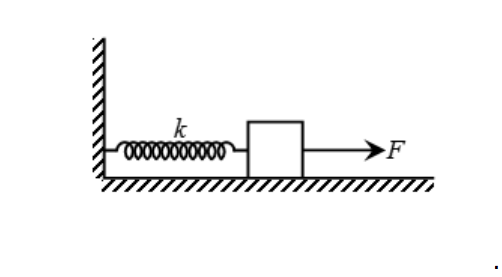here

mass , m = 2.8 kg

amplitude of motion , A = 35 cm

A = 0.35 m

force applied ,F = 927.5 N

let the spring constant be K

F = K * x

927.5 = K * 0.35

K = 2650 N/m

a)

The maximum acceleration of the block , a = F /m

a = 927.5 /2.8 m/s^2 = 331.25 m/s^2

b)

the period of oscillation , T = 2*pi * sqrt(m/K)

T = 2*pi * sqrt(2.8 /2650) s

T = 0.204 s

c)

the angular frequency , w= sqrt(K/m)

w = sqrt(2650 /2.8) = 30.8 rad/s

d)

the speed at equilibrium , vm = A * w

vm = 0.35 * 30.8 m/s = 10.77 m/s

e)

let the velocity be v1 when x1 = 16.2 cm = 0.162 m

using conservation of energy

0.5 * m * vm^2 = 0.5 * m * v1^2 + 0.5 * K * x1^2

2.8 * 10.77^2 = 2.8 * v1^2 + 2650 * 0.162^2

solving for v1

v1 = 9.54 m/s

the velocity is 9.54 m/s

#### Earn Coin

Coins can be redeemed for fabulous gifts.

Similar Homework Help Questions
• ### A block of mass 1.6 ?? is moving across a smooth floor at 13.8 ?/? and...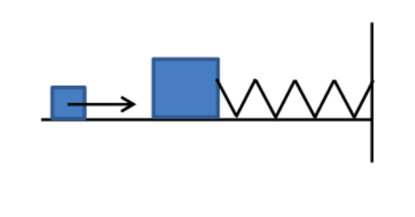A block of mass 1.6 ?? is moving across a smooth floor at 13.8 ?/? and encounters a second block (initially at rest) of mass 3.4 ?? in a fully elastic collision. The second block is attached to a spring of ? = 1250 ?/?. Assume the spring to be massless and does not interfere with the collision. After the collision, the second block is under simple harmonic motion. Determine, a. The amplitude of oscillation. b. The frequency of oscillation....

• ### 7. A block of mass 1.6 kg is moving across a smooth floor at 13.8 m/s...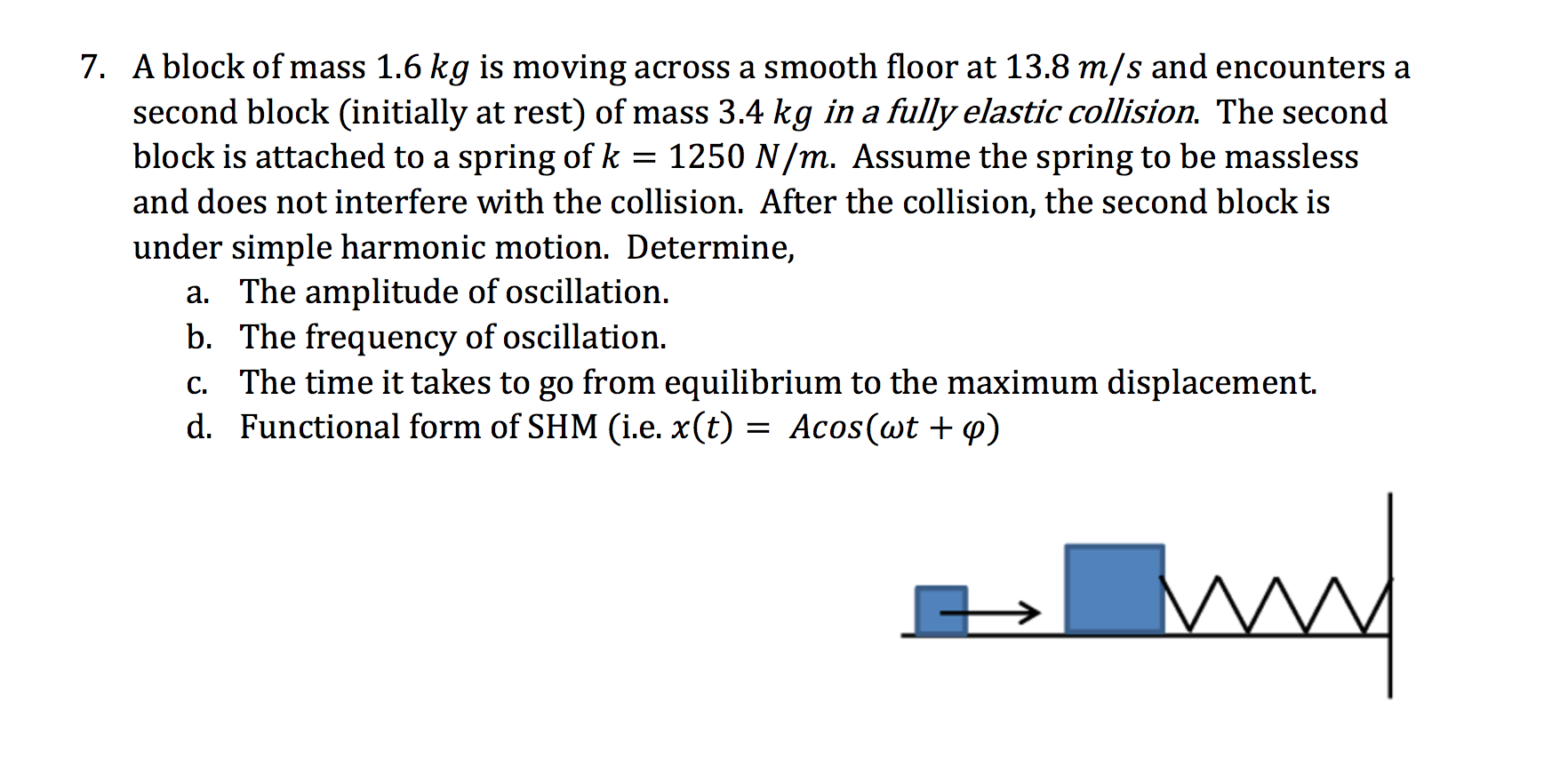7. A block of mass 1.6 kg is moving across a smooth floor at 13.8 m/s and encounters a second block (initially at rest) of mass 3.4 kg in a fully elastic collision. The second block is attached to a spring of k = 1250 N/m. Assume the spring to be massless and does not interfere with the collision. After the collision, the second block is under simple harmonic motion. Determine, a. The amplitude of oscillation b. The frequency of...

• ### Problem3 A (2+0.1y) kg block attached to a spring undergoes simple harmonic motion described by x...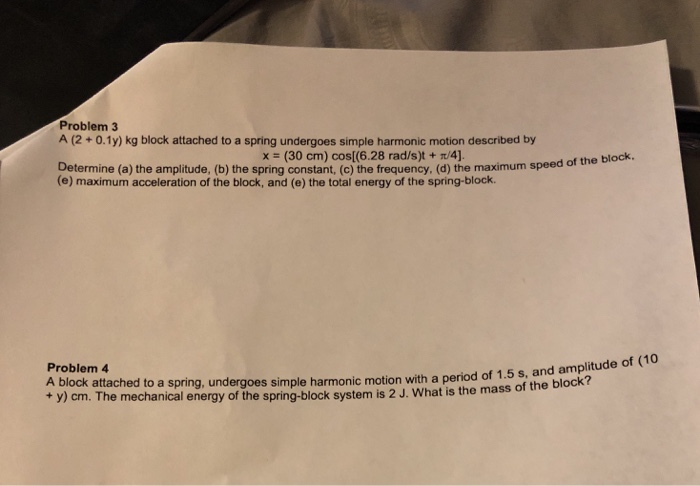Can you please answer both questions, Y=0 Problem3 A (2+0.1y) kg block attached to a spring undergoes simple harmonic motion described by x (30 cm) cos[(6.28 rad/s)t + /4) Determine (a) the amplitude, (b) the spring constant, (c) the frequency, (d) the maximum speed (e) maximum acceleration of the block, and (e) the total energy of the spring-block. of the block Problem 4 A block attached to a spring, undergoes simple harmonic motion with a period of 1.5 + y)...

• ### z waqod A 2- kg block attached to a spring undergoes simple harmonic motion described by...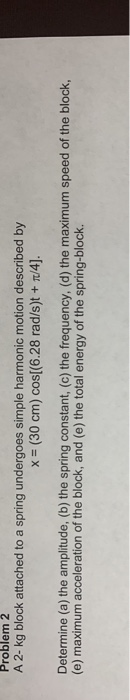z waqod A 2- kg block attached to a spring undergoes simple harmonic motion described by = (30 cm) cos[(6.28 rad/s)t + /4]. Determine (a) the amplitude, (b) the spring constant, (c) the frequency, (d) the maximum speed of the block, (e) maximum acceleration of the block, and (e) the total energy of the spring-block. Problem 3 A block attached to a spring, undergoes simple harmonic motion with a period of 1.5 s, and amplitude of 20 cm. The mechanical...

• ### 2. A block of unknown mass is attached to a spring with a spring constant of...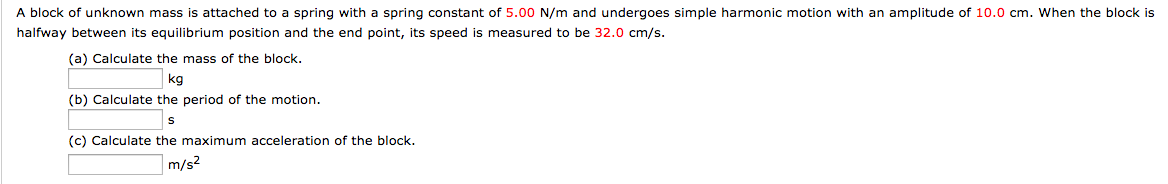2. A block of unknown mass is attached to a spring with a spring constant of 5.00 N/m and undergoes simple harmonic motion with an amplitude of 10.0 cm. When the block is halfway between its equilibrium position and the end point, its speed is measured to be 32.0 cm/s (a) Calculate the mass of the block (b) Calculate the period of the motion (c) Calculate the maximum acceleration of the block. kg m/s

• ### 2. A block of mass M is attached to a wall by a massless spring with...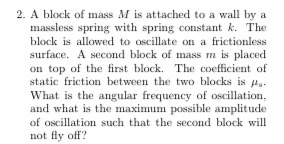2. A block of mass M is attached to a wall by a massless spring with spring constant k. The block is allowed to oscillate on a frictionless surface. A second block of mass m is placed on top of the first block. The coefficient of static friction between the two blocks is his. What is the angular frequency of oscillation, and what is the maximum possible amplitude of oscillation such that the second block will not fly off?

• ### A simple harmonic oscillator consists of a block attached to a spring, moving back and forth...

A simple harmonic oscillator consists of a block attached to a spring, moving back and forth on a frictionless horizontal surface. Suppose the mass of the box is 5.0 kg. The motion is started by holding the box at .50m from its central position, using a force of 40.0 N. Then the box is let go and allowed to perform simple harmonic motion. What is the amplitude of the motion? What is the spring constant k? What is the maximum...

• ### A mass m = 3 kg is attached to a spring with spring constant k =...

A mass m = 3 kg is attached to a spring with spring constant k = 3 N/m and oscillates with simple harmonic motion along the x-axis with an amplitude A = 0.10 m. (a) What is the angular frequency  of this oscillation? (b) What is the period T and the frequency f of the oscillation? (c) If the phase constant  = 0, write down expressions for the displacement, velocity and acceleration of the mass as a function...

• ### A large block P attached to a light spring executes horizontal, simple harmonic motion as it...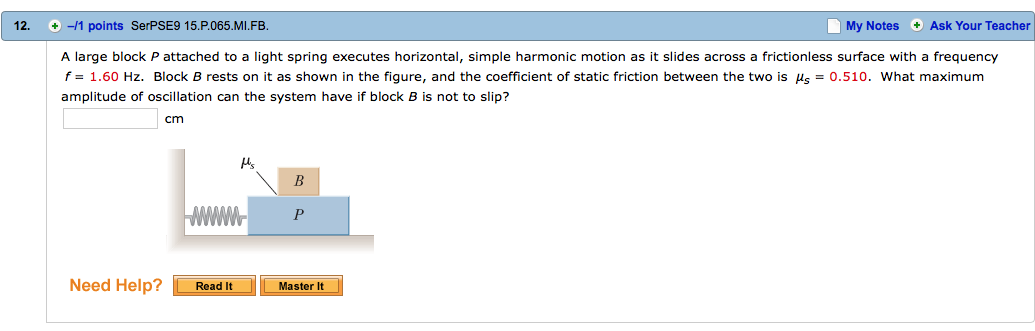A large block P attached to a light spring executes horizontal, simple harmonic motion as it slides across a frictionless surface with a frequency f = 1.60 Hz. Block B rests on it as shown in the figure, and the coefficient of static friction between the two is Mu_s = 0.510. What maximum amplitude of oscillation can the system have if block B is not to slip cm

• ### A simple harmonic oscillator consists of a block attached to a spring, moving back and forth...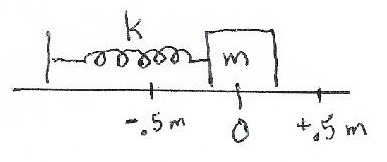A simple harmonic oscillator consists of a block attached to a spring, moving back and forth on a frictionless horizontal surface. Suppose the mass of the box is 5.0 kg. The motion is started by holding the box at 0.50 m from its central position, using a force of 40.0 N. Then the box is let go and allowed to perform simple harmonic motion. (a) What is the amplitude of the motion? (b) What is the spring constant k? (c)...